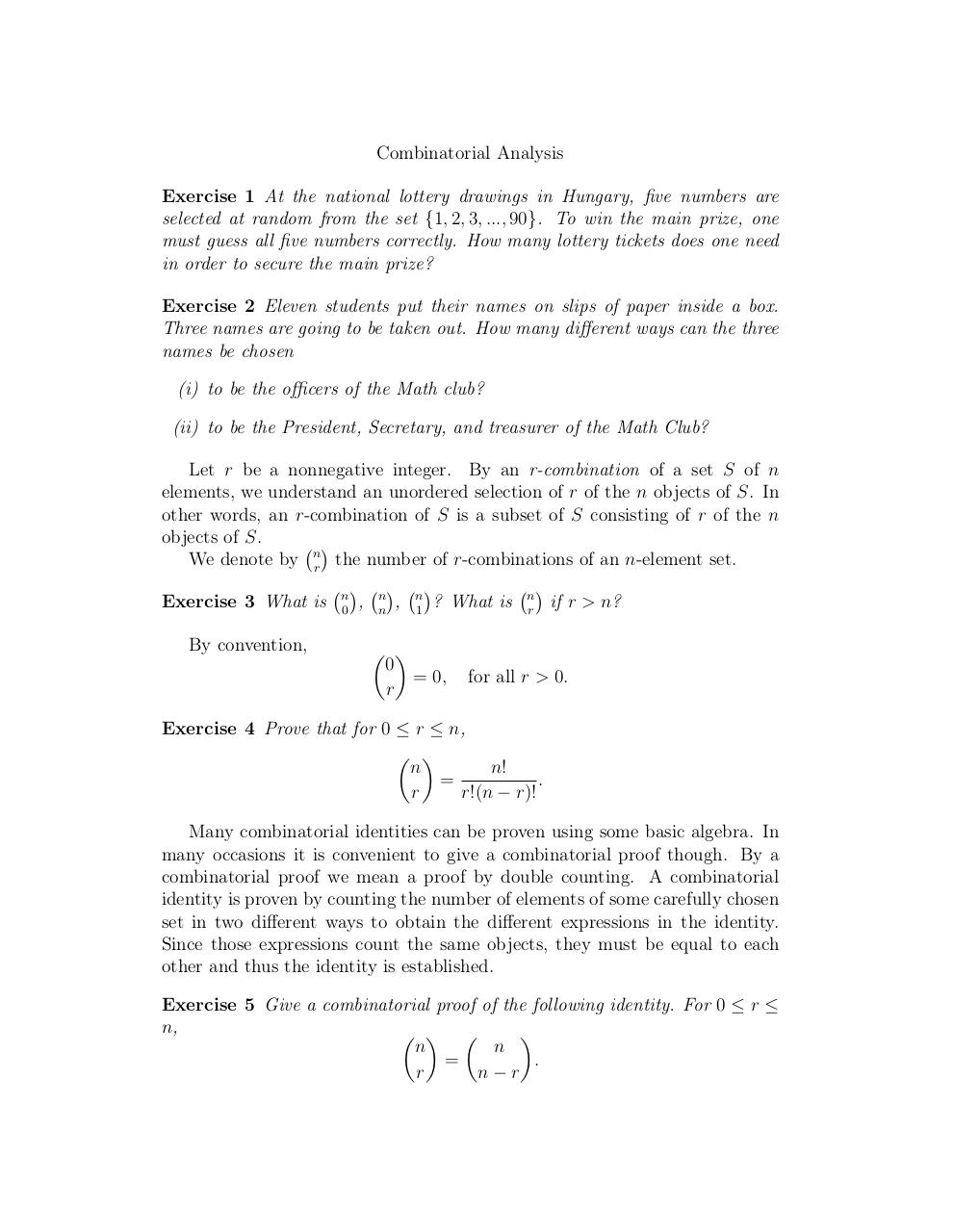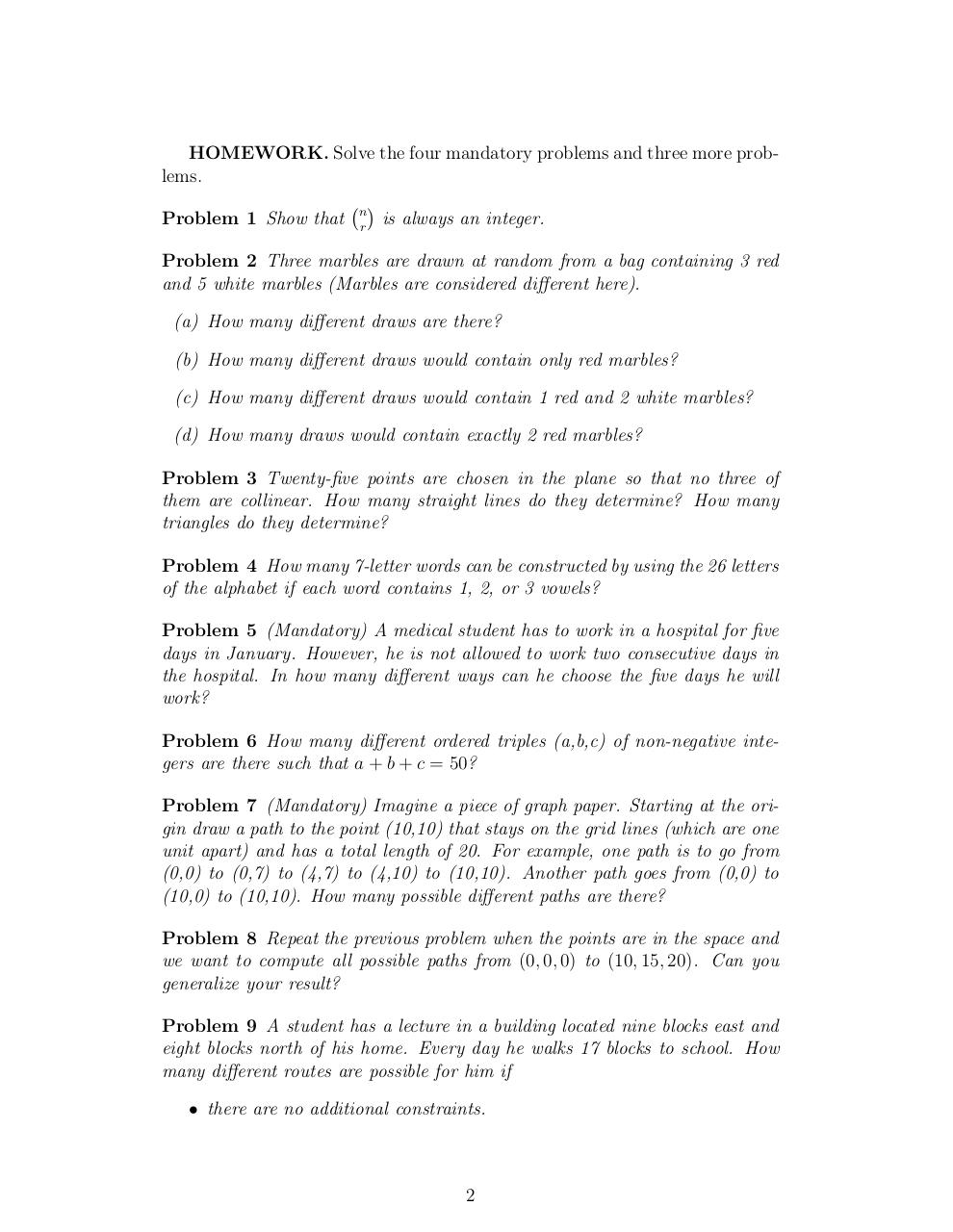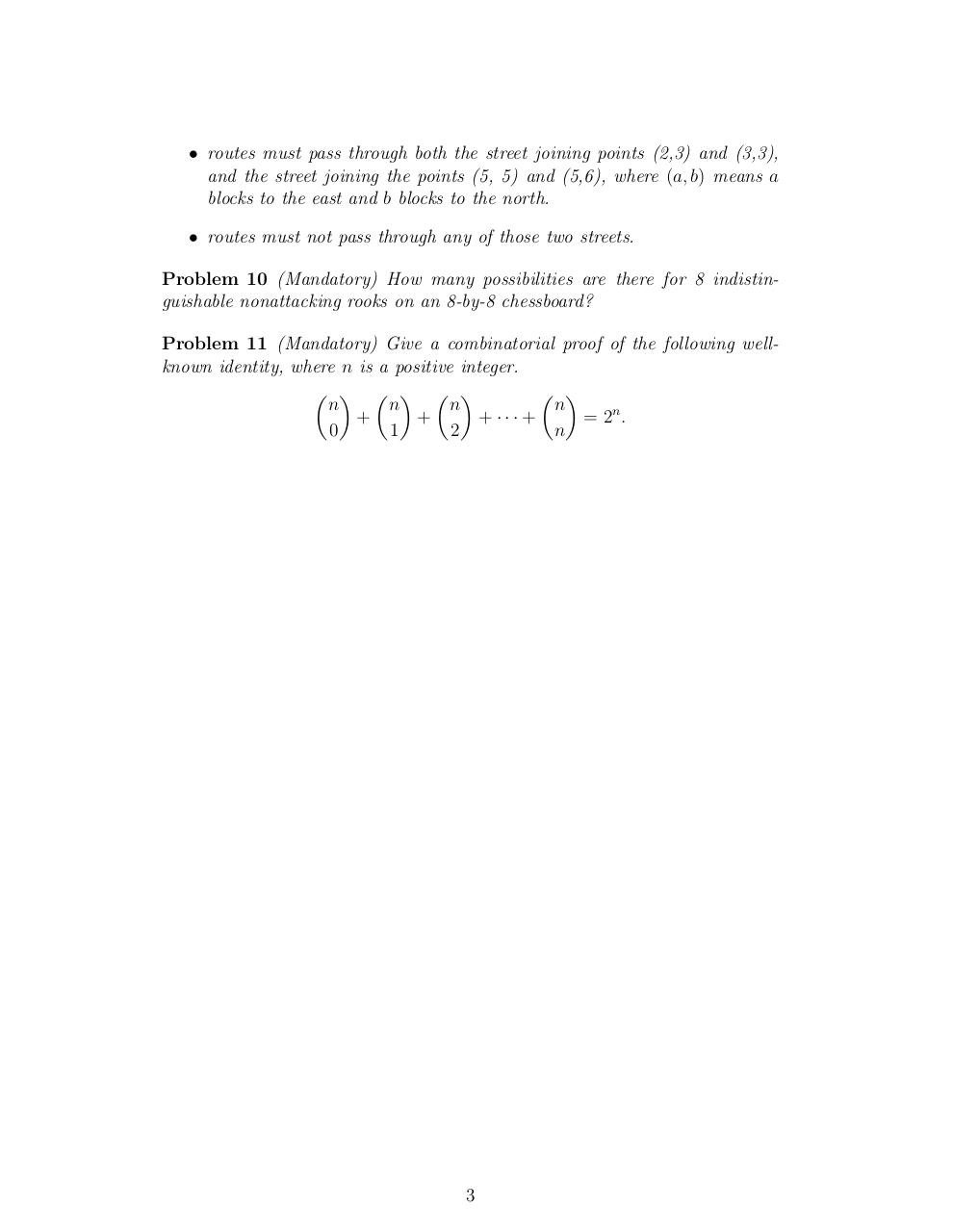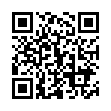# Day 7 combinations without repetition .pdf

### File information

Original filename: Day 7-combinations without repetition.pdf

This PDF 1.4 document has been generated by TeX / pdfTeX-1.40.3, and has been sent on pdf-archive.com on 24/01/2014 at 06:06, from IP address 169.231.x.x. The current document download page has been viewed 652 times.
File size: 98 KB (3 pages).
Privacy: public file

Day 7-combinations without repetition.pdf (PDF, 98 KB)

### Document preview

Combinatorial Analysis
Exercise 1 At the national lottery drawings in Hungary, five numbers are
selected at random from the set {1, 2, 3, ..., 90}. To win the main prize, one
must guess all five numbers correctly. How many lottery tickets does one need
in order to secure the main prize?
Exercise 2 Eleven students put their names on slips of paper inside a box.
Three names are going to be taken out. How many different ways can the three
names be chosen
(i) to be the officers of the Math club?
(ii) to be the President, Secretary, and treasurer of the Math Club?
Let r be a nonnegative integer. By an r-combination of a set S of n
elements, we understand an unordered selection of r of the n objects of S. In
other words, an r-combination of S is a subset of S consisting of r of the n
objects of S.

We denote by nr the number of r-combinations of an n-element set.




Exercise 3 What is n0 , nn , n1 ? What is nr if r &gt; n?
By convention,
 
0
= 0,
r

for all r &gt; 0.

Exercise 4 Prove that for 0 ≤ r ≤ n,
 
n
n!
.
=
r!(n − r)!
r
Many combinatorial identities can be proven using some basic algebra. In
many occasions it is convenient to give a combinatorial proof though. By a
combinatorial proof we mean a proof by double counting. A combinatorial
identity is proven by counting the number of elements of some carefully chosen
set in two different ways to obtain the different expressions in the identity.
Since those expressions count the same objects, they must be equal to each
other and thus the identity is established.
Exercise 5 Give a combinatorial proof of the following identity. For 0 ≤ r ≤
n,
  

n
n
=
.
r
n−r

HOMEWORK. Solve the four mandatory problems and three more problems.

Problem 1 Show that nr is always an integer.
Problem 2 Three marbles are drawn at random from a bag containing 3 red
and 5 white marbles (Marbles are considered different here).
(a) How many different draws are there?
(b) How many different draws would contain only red marbles?
(c) How many different draws would contain 1 red and 2 white marbles?
(d) How many draws would contain exactly 2 red marbles?
Problem 3 Twenty-five points are chosen in the plane so that no three of
them are collinear. How many straight lines do they determine? How many
triangles do they determine?
Problem 4 How many 7-letter words can be constructed by using the 26 letters
of the alphabet if each word contains 1, 2, or 3 vowels?
Problem 5 (Mandatory) A medical student has to work in a hospital for five
days in January. However, he is not allowed to work two consecutive days in
the hospital. In how many different ways can he choose the five days he will
work?
Problem 6 How many different ordered triples (a,b,c) of non-negative integers are there such that a + b + c = 50?
Problem 7 (Mandatory) Imagine a piece of graph paper. Starting at the origin draw a path to the point (10,10) that stays on the grid lines (which are one
unit apart) and has a total length of 20. For example, one path is to go from
(0,0) to (0,7) to (4,7) to (4,10) to (10,10). Another path goes from (0,0) to
(10,0) to (10,10). How many possible different paths are there?
Problem 8 Repeat the previous problem when the points are in the space and
we want to compute all possible paths from (0, 0, 0) to (10, 15, 20). Can you
Problem 9 A student has a lecture in a building located nine blocks east and
eight blocks north of his home. Every day he walks 17 blocks to school. How
many different routes are possible for him if
• there are no additional constraints.

2

• routes must pass through both the street joining points (2,3) and (3,3),
and the street joining the points (5, 5) and (5,6), where (a, b) means a
blocks to the east and b blocks to the north.
• routes must not pass through any of those two streets.
Problem 10 (Mandatory) How many possibilities are there for 8 indistinguishable nonattacking rooks on an 8-by-8 chessboard?
Problem 11 (Mandatory) Give a combinatorial proof of the following wellknown identity, where n is a positive integer.
     
 
n
n
n
n
+
+
+ ··· +
= 2n .
0
1
2
n

3#### HTML Code

Copy the following HTML code to share your document on a Website or Blog

#### QR Code### Related keywords